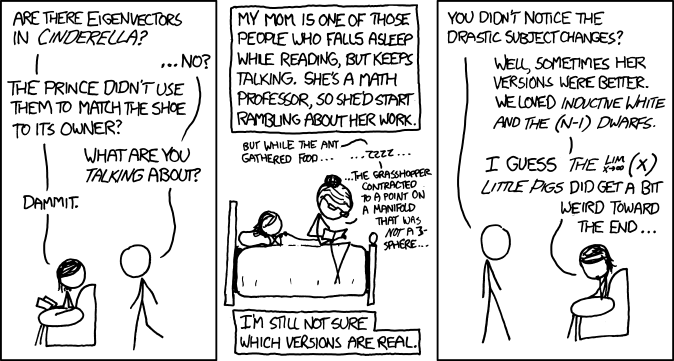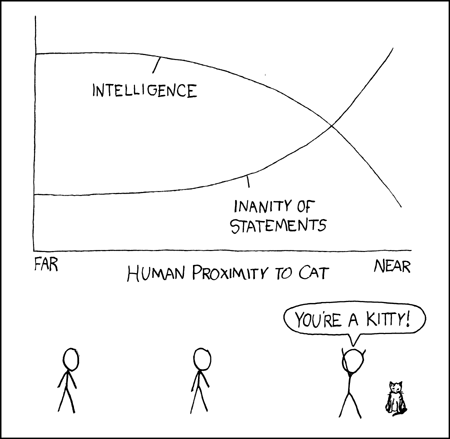Sep 22

## Derivatives and Rate of Change

Here are two problems I used in my calculus 1 class, just after teaching them the definition of the derivative and discussing average and instantaneous rates of change.

1. My car has a digital read out with the average fuel economy.  I used to have a car which also had the instantaneous fuel economy.What is the difference between these two fuel economies?  Under what circumstances is each more useful?  How can I use the average fuel economy feature to approximate my instantaneous fuel economy?  (Your answer should be  2-5 complete sentences).
You can use this QR code to access it1. What is the average rate of change of the mass of Antarctica’s ice?
2. What is the average rate of change of the mass of Greenland’s ice?
3. Looking at the graphs, do you think it is reasonable to talk about the instantaneous rate of change for these quantities?  Why or why not?
Then answer the following questions. Give all answers in complete sentences with units.

Jul 27

## Homelessness

Here’s a warm up activity to get students thinking mathematically and thinking about what math is:

One of my friends posted this picture on Facebook.  Use it and whatever other resources you need to answer the following questions.a) Assuming the first statement is correct, is the second statement correct?

b)  What (if anything) is wrong with this “solution” to homelessness?

c) To what extent was this problem “doing math”?  Were both parts a and b math problems?

Apr 13

## Limits and Fairy Tales

Posted in Comic Strips,

I asked my calculus students to read this xkcd strip from http://xkcd.com/872/a) What is $\lim_{x\rightarrow \infty} (x )$?
b) Explain what might have been weird about the $\lim_{x\rightarrow \infty} (x )$ little pigs.

Mar 23

## Cat Proximity and its effect on the human intelect

Posted in Comic Strips,

Randall Munroe drew a lovely comic strip graphing the effects of proximity to a cat on the human intellect:(You can view it here: http://xkcd.com/231/).

In differential calculus I asked students to describe the graphs using their knowledge of calculus.  Statements about both the first and second derivative are expected.

In Intermediate Algebra here at UDM we study qualitative graphs, so I also asked my Algebra students to describe the graph.  These students have to say things like the intelligence of the human is decreasing, but at an ever faster rate.

One caution with this comic: some students do not know what “inanity” means, so I use this problem on the review sheet rather than the exam.

Dr. Dawn's blog